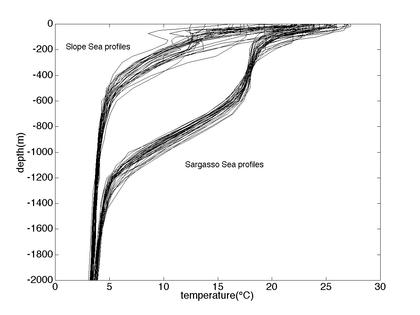Velocity and deformation scales

 The scale-widths obtained from the velocity data agree quite well with estimates of the local radius of deformation computed from hydrographic profiles taken just outside the stream to either side, Figure 9 (below).Figure 9. Temperature profiles from 30 hydrographic stations to either side of the Gulf Stream in the immediate vicinity of the Oleander line. These and the corresponding salts were used to calculate the radius of deformation. To see this we use linear theory for planetary wave motion in a stratified ocean. Essentially, we look for the lowest eigenvalue (i=1) for the equation: d2w/dz2 + ci2N2w=0 where N is the Brunt-Vailala frequency, ci are the eigenvalues, and the subscript i refers to mode number. The radius of deformation = 1/cif in meters where f = Coriolis parameter = 0.9x10-4s-1 for this region of the Gulf Stream. Using a set of 30 hydrographic stations near the Oleander line just north and south of the Gulf Stream we obtain 19.7 and 34.3 km respectively. These values agree well with estimates made for larger 4° by 4° regions in the vicinity of the Gulf Stream (Emery et al., 1984). These values also agree quite well with the scale-widths for the mean velocity shear determined from the stream-coordinate mean (Figure 2).